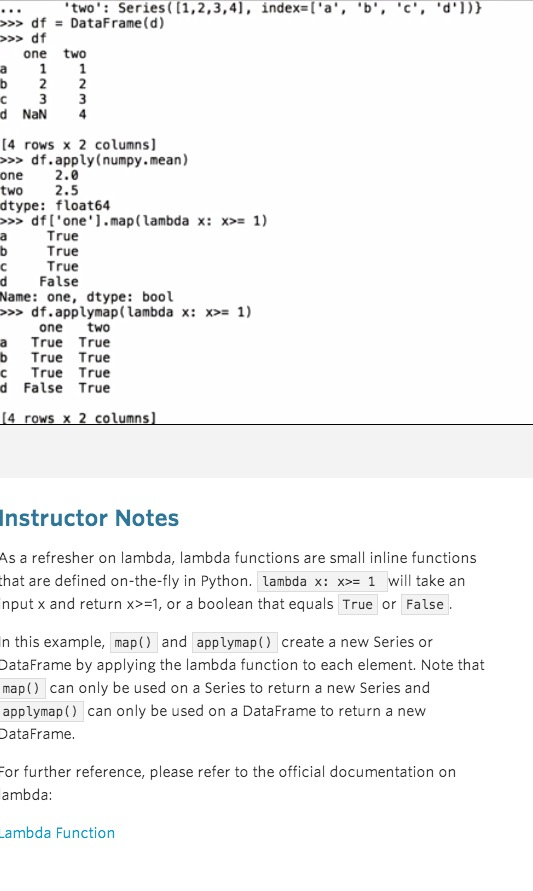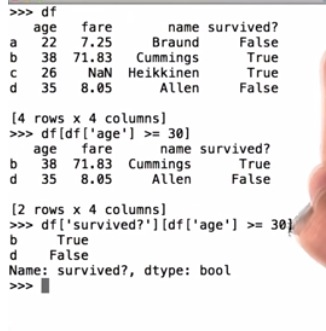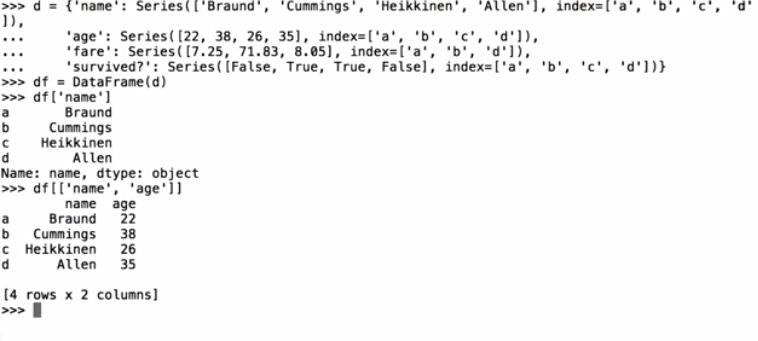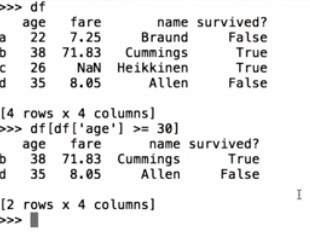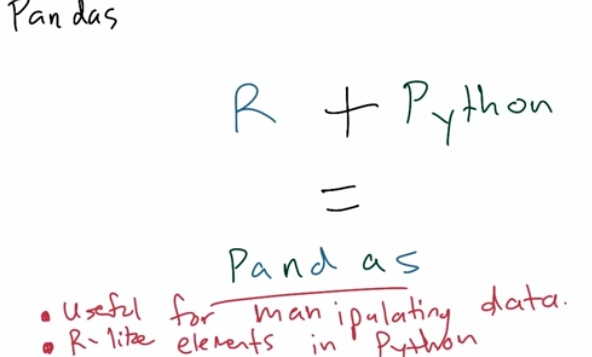• R working best for manipulating data
• Take the best elements of R and implement it in Python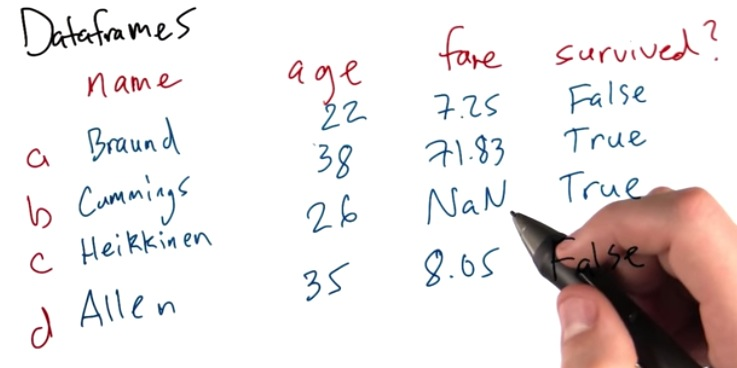• Often R and dataset has Dataframes Structure
• Consist of 2D graph
• Can be various datatypes, string, int, float, or boolean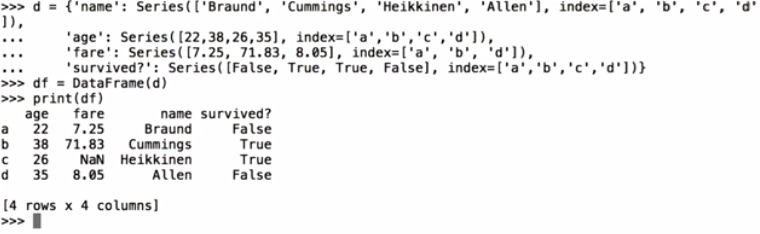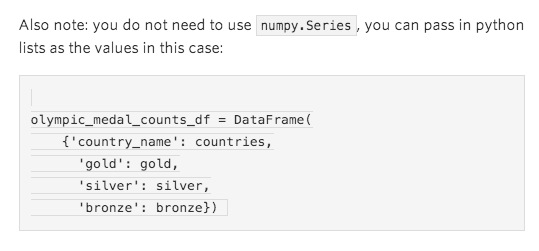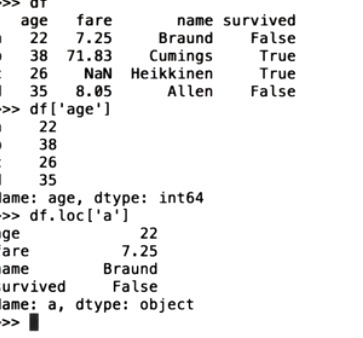• This is the dataframe command line
• We can find the examples based on dictionary, like provided key "age" returning examples just based on age
• Or maybe trying to find the examples "a", which return all the correlated data of a id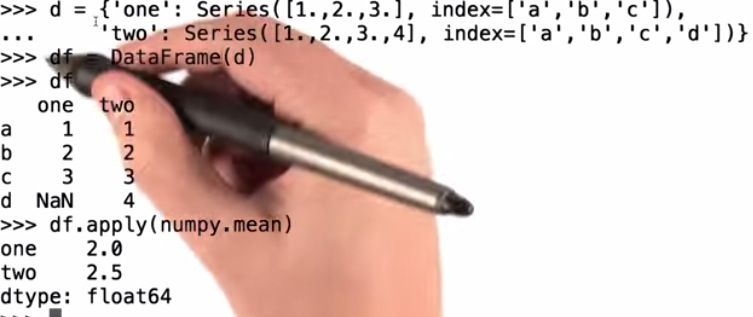• Here we are creating the dataframes, with value based on index 'abcde'
• We can apply the mean of the data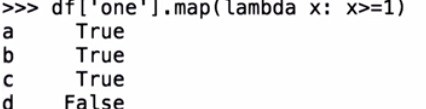• We can map the data of 'one' that meet the lambda requirements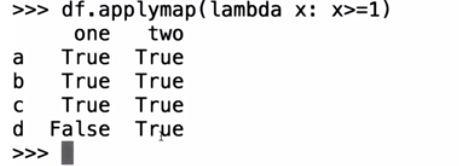• Or apply the whole data, mapping with spesific lambda requirement
• Next, How to obtain the data that we want to handle